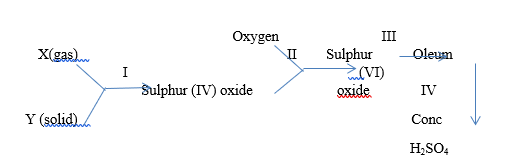# (a) The following reaction scheme is an illustration of the contact process. Study the scheme and answer the questions that follow. (i) Name X and Y (ii) Wr...

Question 1

(a) The following reaction scheme is an illustration of the contact process. Study the scheme and answer the questions that follow.(i) Name X and Y

(ii) Write a balanced chemical equation for each of the processes I, II, III and IV

(iii) Name the catalyst used in process II

(iv) Using Le Chatelier's principle, explain briefly why increasing the temperature would not favour the reaction in II

(v) State two uses of SO$$_2$$

(b) Consider the following equation: 2H$$_{2(g)}$$ + O$$_{2(g)}$$ $$\to$$ 2H$$_2$$O$$_{(g)}$$

Calculate the volume of unused oxygen gas when 40 cm$$^3$$ of hydrogen gas is sparked with 30cm$$^3$$ of oxygen gas

(c) Calcium carbonate of mass 1.0 g was heated until there was no further change.

• Write an equation for the reaction which took place.
• Calculate the mass of the residue.
• Calculate the volume of the gas evolved at s.t.p.
• What would be the volume of the gas measured at 15  and 760 mm Hg?  [C= 12.0, O = 16.0, Ca = 40.0, molar volume of a gas at s.t.p. = 22.4 dm$$^3$$ ]